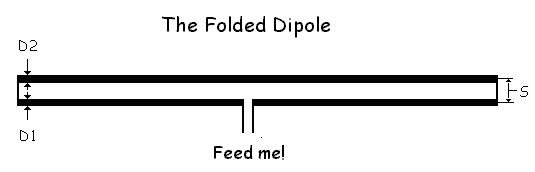Feeding a Folded Dipole Antenna

If you play with the numbers, and get the input impedance down to 200ohms, you'll need a 4:1 balun to feed it from 50 ohm coax
post 01 Jan 2022160

Loop antenna resonant frequency calculator
The simple calculator below uses the well-known standard formula for determining the total wire length in feet for a single element

Logarithmic Periodic Dipole Antenna Calculator
The javascript starts with the minimum value of = 0.76. If a solution could be found, is increased as long as the length of the boom

Magnetic Loop Antenna Calculator
Magnetic loops or mag loops, can give surprisingly good performance when they are carefully designed and constructed

Parabolic dish calculator
Complete all white boxes and then click the calculate button to obtain results in the green boxes